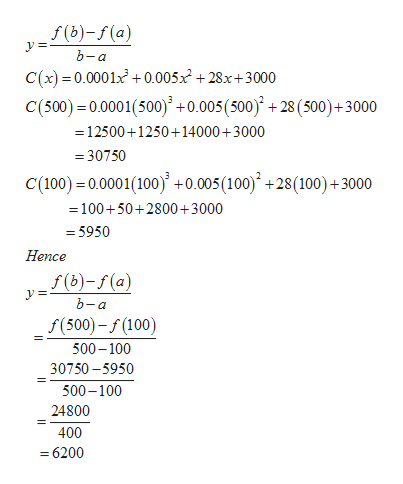# Suppose total cost in dollars from the production of x printers is given byC(x) = 0.0001x3 + 0.005x2 + 28x + 3000 (a) Find the average rate of change of total cost when production changes from 100 to 500 printers. (b) Find the average rate of change of total cost when production changes from 500 to 700 printers.

Question
44 views

Suppose total cost in dollars from the production of x printers is given by

C(x) = 0.0001x3 + 0.005x2 + 28x + 3000

(a) Find the average rate of change of total cost when production changes from 100 to 500 printers.

(b) Find the average rate of change of total cost when production changes from 500 to 700 printers.
check_circle

Step 1

Suppose total cost in dollar from the production of x printers is given by

C(x) = 0.0001x3+0.005x2+28x+3000

Find the average rate f change of total cost when production changes

1. a) from 100 to 500 printers
2. b) from 500 to 700 printer
Step 2

The average  rate of change of a function

Step 3

Given

from 100 to 500...help_outlineImage Transcriptionclosef(b)-f(a) b-a C(x) 0.0001x + 0.005x2 +28x+3000 C(500) 0.0001(500)+0.005 (500) + 28 (500)+3000 12500+1250+14000+3000 30750 C(100) 0.0001(100) +0.005(100) +28(100)+3000 = 100+50+2800+3000 = 5950 Hence f(b)-f(a) У b-а f(500)-f(100) 500-100 30750-5950 500-100 24800 400 6200 fullscreen

### Want to see the full answer?

See Solution

#### Want to see this answer and more?

Solutions are written by subject experts who are available 24/7. Questions are typically answered within 1 hour.*

See Solution
*Response times may vary by subject and question.
Tagged in

### Calculus